PSABDVPY - Grade 4 Mathematics Practice Test 2014

Talia used 672 charms to make key chains for the school fair. She used 7 charms for each key chain she made. What was the total number of key chains Talia made for the school fair?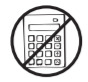Select one:

A string is cut into 10 equal parts. Which of the following shows two ways to represent 1 part of the string?Select one:

Makara wrote the equation shown belowWhat number belongs in the ? to make Makara’s equation true?Select one:

Draw your quadrilateral on paper, take a picture, and upload it using the upload icon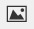If you do not have the ability to upload an image of your work, type "drawing is on paper."Part A)

Jean putcup of yogurt on top of each bowl of fruit she made. She made 10 bowls of fruit.

a. Write an expression that shows the amount, in cups, of yogurt that Jean put on all 10 bowls of fruit.Part B)

b. In your Student Answer Booklet, draw a fraction model to show the amount, in cups, of yogurt that Jean put on all 10 bowls of fruit.

Draw your graph on paper, take a picture, and upload it using the upload iconIf you do not have the ability to upload an image of your work, type "Graph is on paper."Part C)

Each bowl Jean made containedcup of fruit.

c. What is the total number of cups of fruit in all 10 bowls Jean made? Write your answer as a mixed number. Show your work.Andy measured the lengths of seeds from different plants. The lengths, in inches, of the seeds Andy measured are shown in the table below.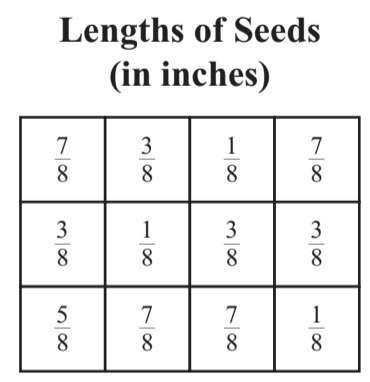Which of these line plots correctly shows the information in the table?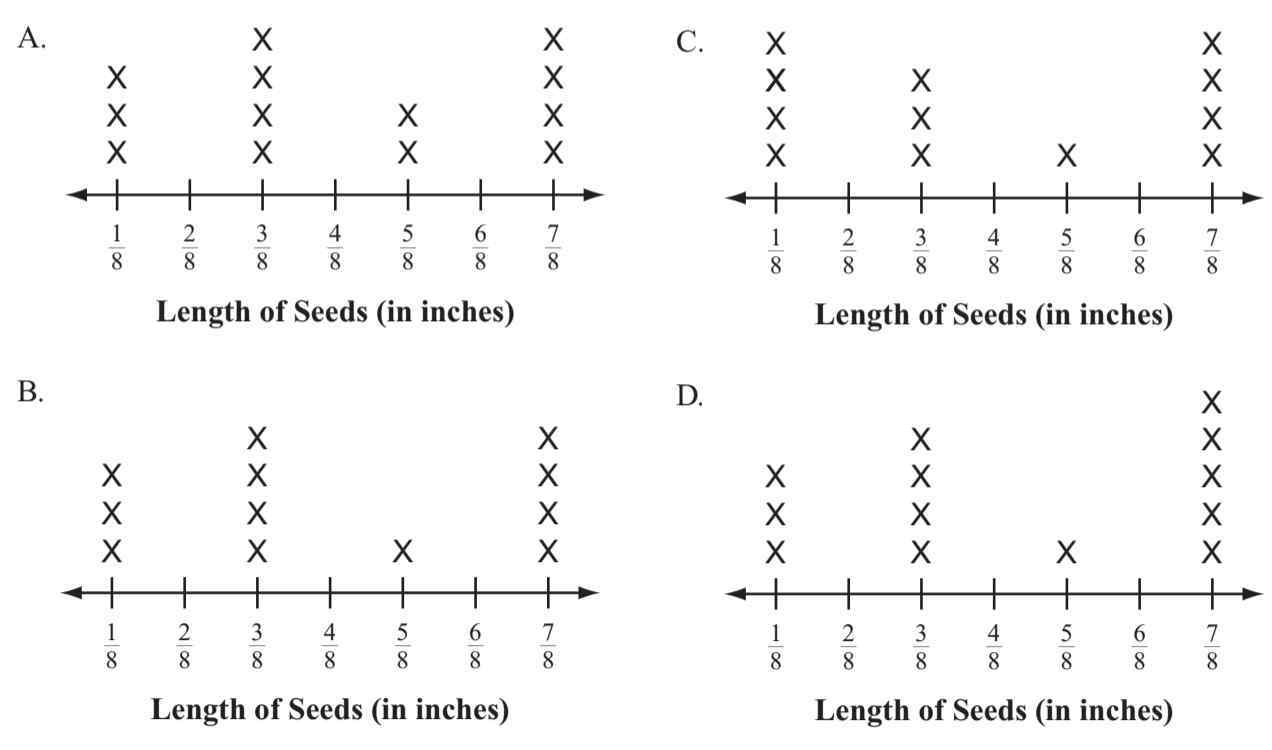Select one:

Which of these is equivalent to 68,051?Select one:

Which of these equations shows that 63 is 9 times as many as 7?Select one:

Lee wrote the equation shown below.What number belongs in the ? to make Lee’s equation true?Select one:

The angle below measures 90°.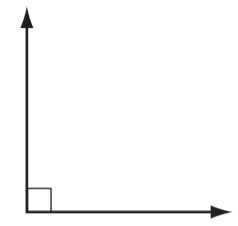Which of the following angles measures about 100°?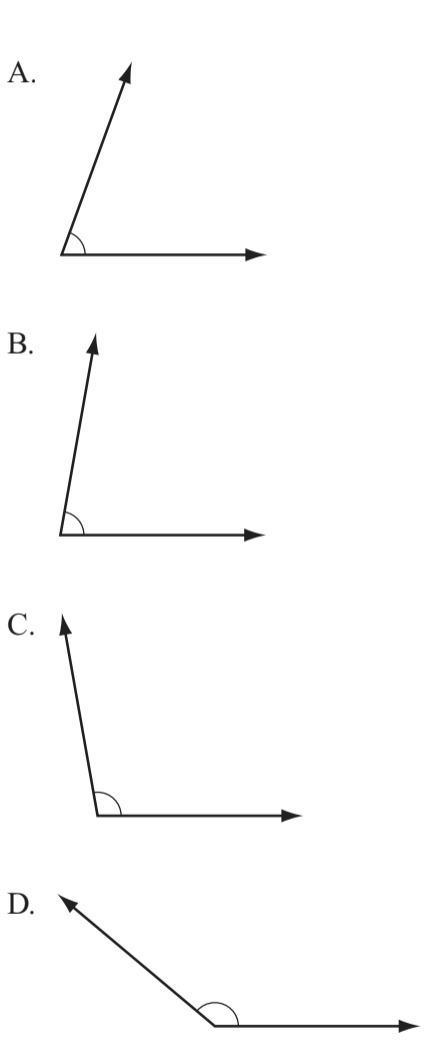Select one:
Part A)

Aaron shaded squares to make a pattern of T shapes, as shown below.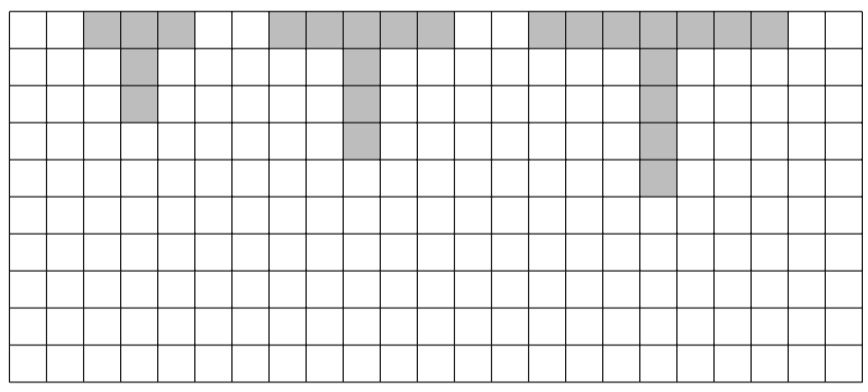Aaron shaded 5 squares to make the first T. Then he shaded 3 more squares each time he made the next T in his pattern. Aaron continued his pattern.

a. On the grid in your Student Answer Booklet, shade squares to make the fourth T in Aaron’s pattern.

Draw your graph on paper, take a picture, and upload it using the upload iconIf you do not have the ability to upload an image of your work, type "Graph is on paper."Part B)

b. How many squares in all does Aaron need to shade to make the sixth T in his pattern? Show or explain how you got your answer.Type your answer below as a number (example: 5, 3.1, 4 1/2, or 3/2):
Part C)

c. Will there be any T in Aaron’s pattern that has exactly 30 shaded squares? Show or explain how you got your answer.Cho drew a shape. The angles inside Cho’s shape are all obtuse angles. Which of the following could be the shape that Cho drew?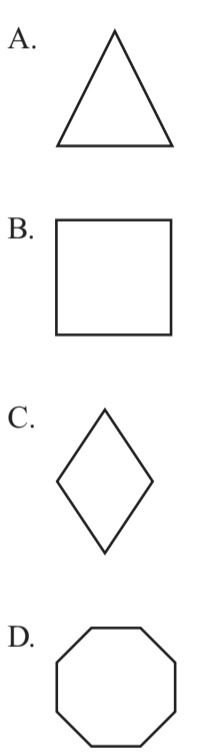Select one:

Which of these shows shape O with one of its lines of symmetry?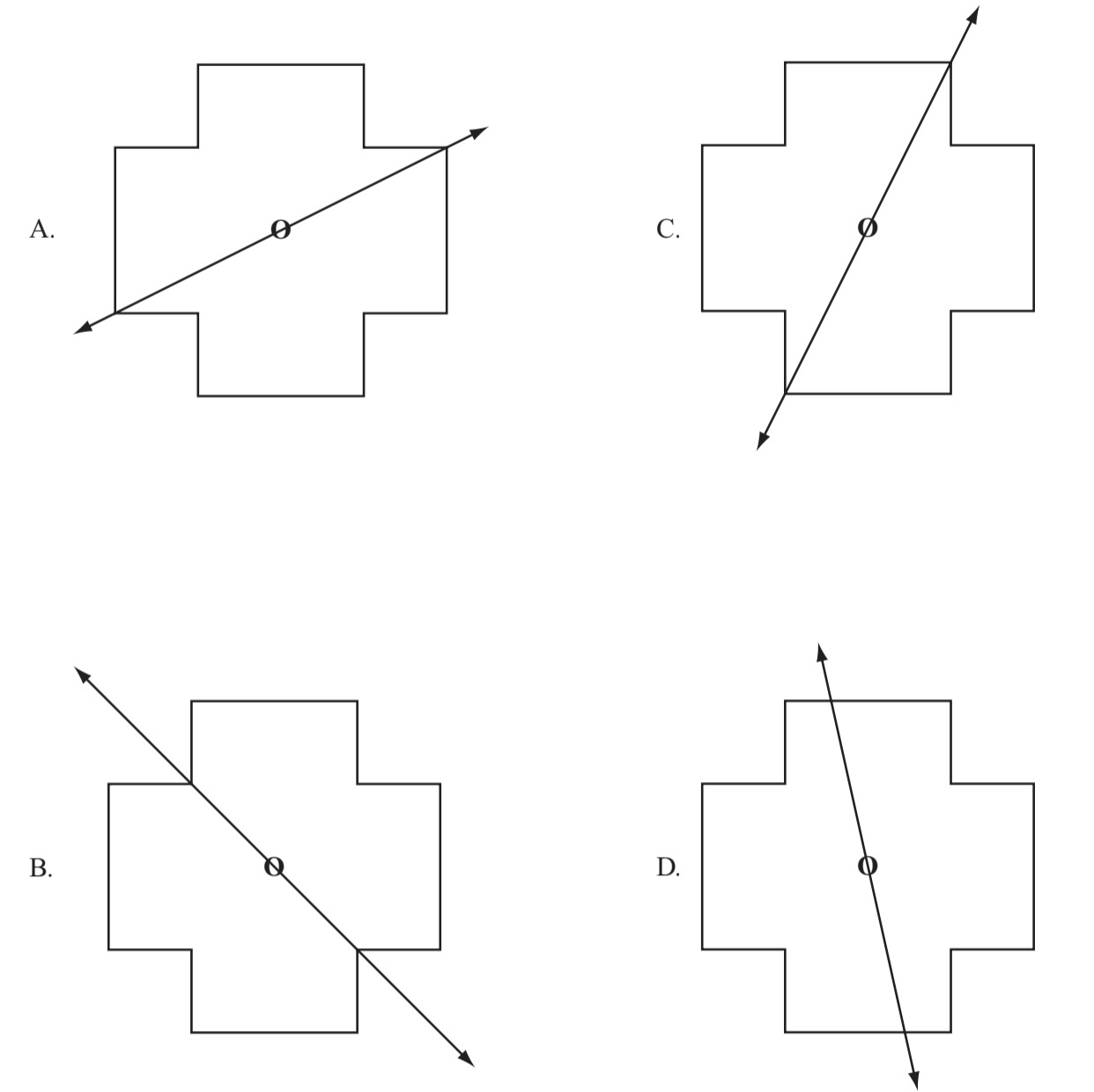Select one:

Corn muffins cost \$2 each. Blueberry muffins cost \$3 each. Which of the following equations can be used to find m, the total cost in dollars of 8 corn muffins and 7 blueberry muffins?Select one:

Angle XYZ is a 180° angle. Angle XYZ is divided into three smaller angles, as shown below.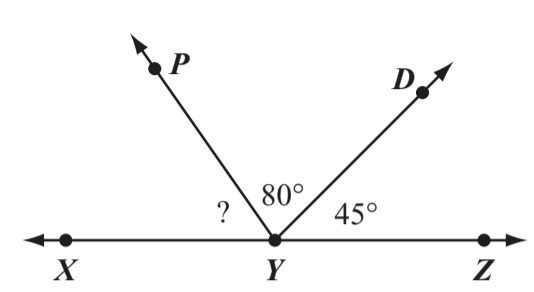What is the measure of angle XYP?Select one:

On Monday, it took Aliza 30 minutes to do her homework and 45 minutes to do her chores. What was the total amount of time Aliza spent doing homework and chores on Monday?Select one:

Which of the following best represents a pair of perpendicular lines?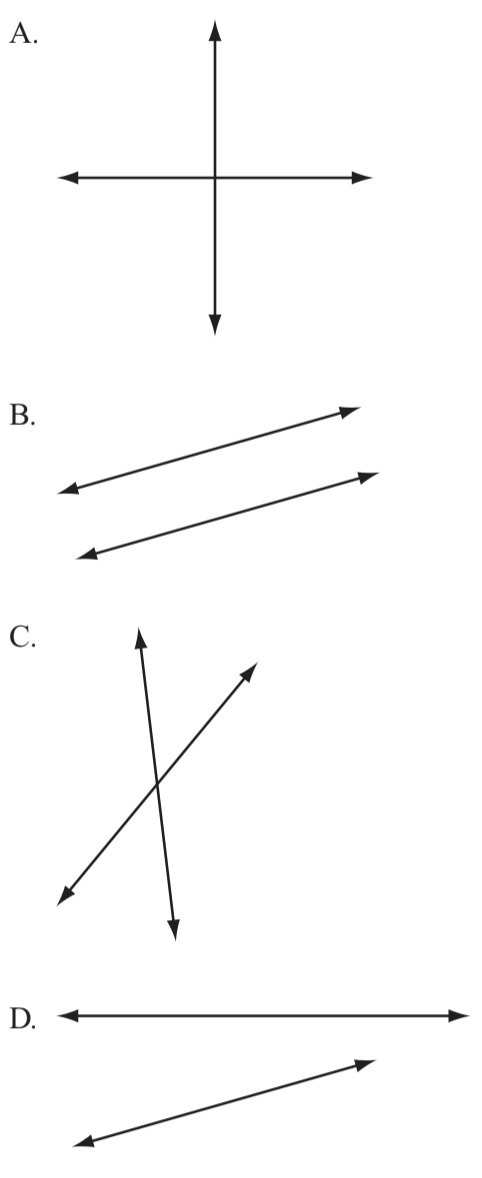Select one:

Shanti has a pear that weighs 0.28 pound. Taylor has a pear that weighs 0.32 pound. Write a number sentence to compare the weights of the two pears. Use one of these symbols, >, <, or =, to compare the numbers in your number sentence.Kiril’s clues about a number are shown in the box below.

• It is an even number.
• It is a multiple of 5.
• It is less than 28.

Write a number that fits all of Kiril’s clues.Type your answer below as a number (example: 5, 3.1, 4 1/2, or 3/2):

Which of these is true?Select one:

An angle and a protractor are shown below.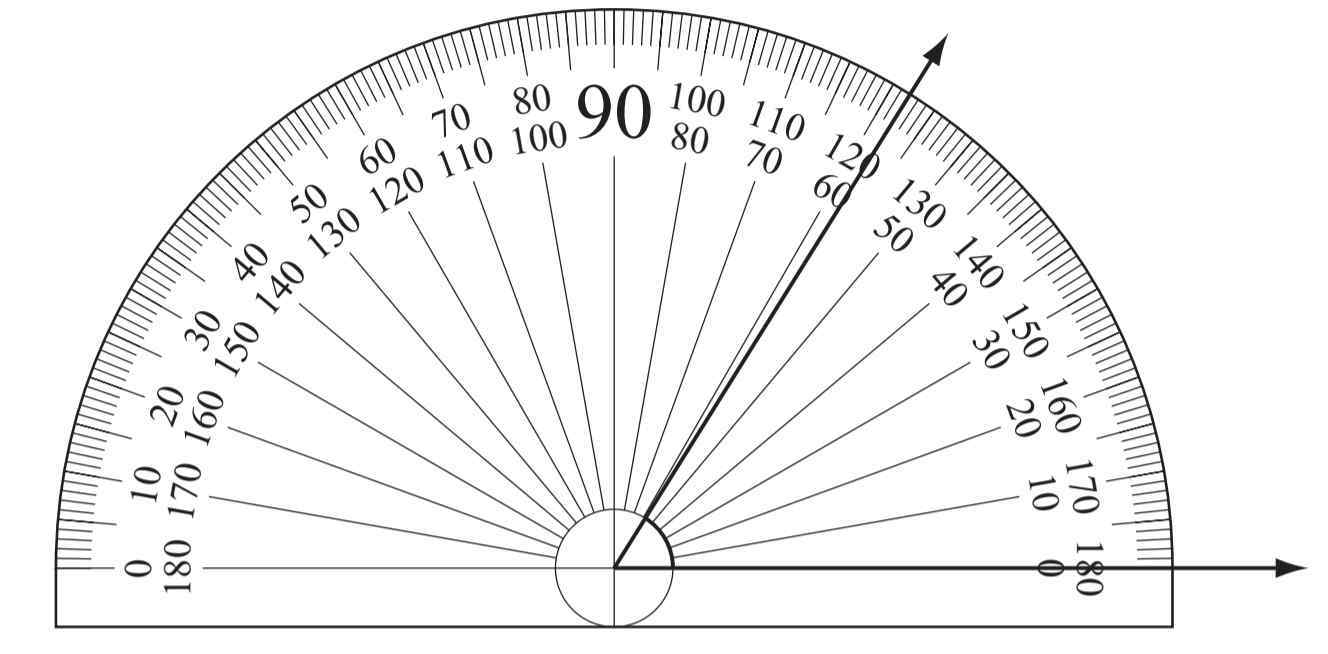What is the measure of the angle?Select one: